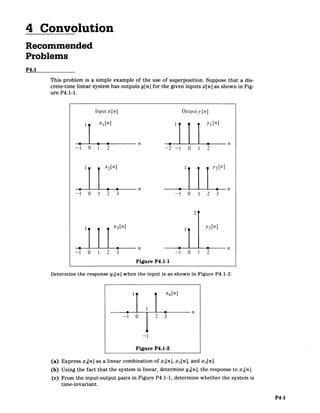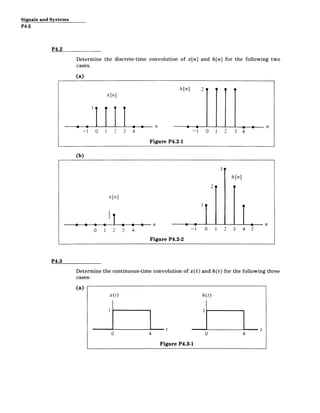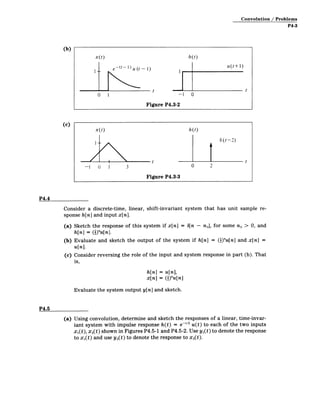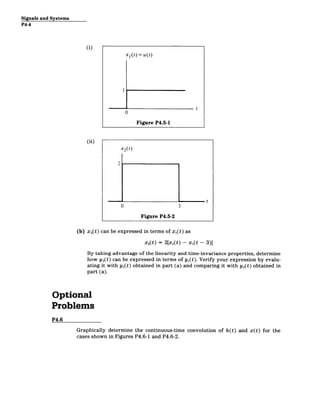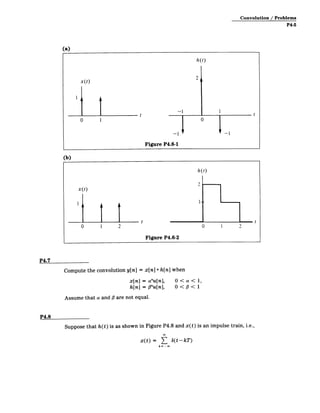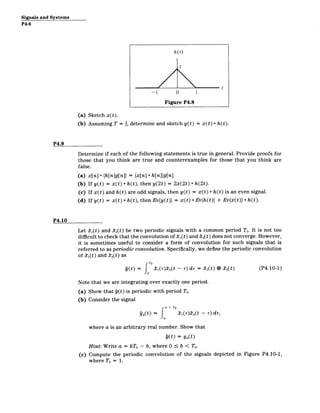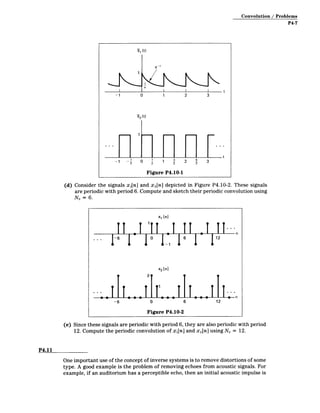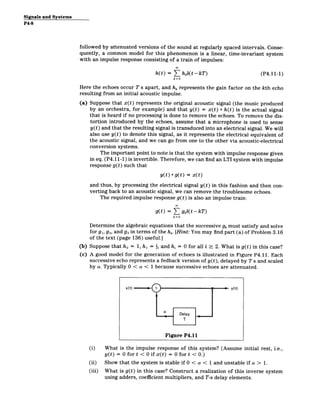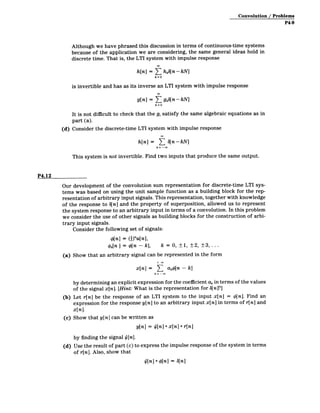Nächste SlideShareMatlab Assignment Help
1 von 10Matlab Assignment HelpMatlab Assignment ExpertsSignal Processing Assignment HelpMatlab Assignment ExpertsSignal and Systems part iPatrickMumba7P3.4,p3.2saumyachaturvedi91Signals Processing Homework HelpMatlab Assignment ExpertsSignals and systems: Part iiPatrickMumba7

### Convolution problems

1. 4 Convolution Recommended Problems P4.1 This problem is a simple example of the use of superposition. Suppose that a dis­ crete-time linear system has outputs y[n] for the given inputs x[n] as shown in Fig­ ure P4.1-1. Input x[n] Outputy [n] III xn] yi[n] 0 0 n 0 n -1 0 1 2 -2 -1 0 1 2 x2[n] 1 2[n] 0 0 n 0 0 n -1 0 1 2 3 -1 0 1 2 3 2 1 1 X3 [n] y3[n] .-. n -n -1 0 1 2 3 -1 0 1 2 Figure P4.1-1 Determine the response y4 [n] when the input is as shown in Figure P4.1-2. I x4[n] -1 0 2 3 012 -1 Figure P4.1-2 (a) Express x 4[n] as a linear combination of x 1[n], x 2[n], and x 3[n]. (b) Using the fact that the system is linear, determine y4[n], the response to x 4[n]. (c) From the input-output pairs in Figure P4.1-1, determine whether the system is time-invariant. P4-1
2. Signals and Systems P4-2 P4.2 Determine the discrete-time convolution of x[n] and h[n] for the following two cases. (a) h[n] 2 x[n] 0 .11 111 11J 00 Fiur P42- -1 0 1 2 3 4 -1 0 1 2 3 4 Figure P4.2-1 (b) 3 h[nI 2 x [n ] X17 0 1 2 3 4 -1 0 1 2 3 4 5 Figure P4.2-2 P4.3 Determine the continuous-time convolution of x(t) and h(t) for the following three cases: (a) x(t) h(t) t t 0 4 0 4 Figure P4.3-1
3. Convolution / Problems P4-3 (b) x(t) h (t) 1-- -(t- 1) u (t - 1) u(t+1 t t 0 1 -1 0 Figure P4.3-2 (c) x(t) h (t) F6(t-2) -l 0 1 3 0 2 Figure P4.3-3 P4.4 Consider a discrete-time, linear, shift-invariant system that has unit sample re­ sponse h[n] and input x[n]. (a) Sketch the response of this system if x[n] = b[n - no], for some no > 0, and h[n] = (i)"u[n]. (b) Evaluate and sketch the output of the system if h[n] = (I)"u[n] and x[n] = u[n]. (c) Consider reversing the role of the input and system response in part (b). That is, h[n] = u[n], x[n] = (I)"u[n] Evaluate the system output y[n] and sketch. P4.5 (a) Using convolution, determine and sketch the responses of a linear, time-invar­ iant system with impulse response h(t) = e-t 2 u(t) to each of the two inputs x1(t), x2(t) shown in Figures P4.5-1 and P4.5-2. Use yi(t) to denote the response to x1(t) and use y2(t) to denote the response to x2(t).
4. Signals and Systems P4-4 (i) X1(t) = u(t) t 0 Figure P4.5-1 (ii) x2(t) 2 t 0 3 Figure P4.5-2 (b) x 2(t) can be expressed in terms of x,(t) as x 2(t) = 2[x(t) - xi(t - 3)] By taking advantage of the linearity and time-invariance properties, determine how y 2(t) can be expressed in terms of yi(t). Verify your expression by evalu­ ating it with yl(t) obtained in part (a) and comparing it with y 2(t) obtained in part (a). Optional Problems P4.6 Graphically determine the continuous-time convolution of h(t) and x(t) for the cases shown in Figures P4.6-1 and P4.6-2.
5. Convolution / Problems P4-5 (a) h(t) x(t) 2 0 1 t 0 t1 (b) Figure P4.6-1 h (t) x(t) 0 1 2 t Figure P4.6-2 0 1 2 t P4.7 Compute the convolution y[n] = x[n] * h[n] when x[n] =au[n], O< a< 1, h[n] =#"u[n], 0 < #< 1 Assume that a and # are not equal. P4.8 Suppose that h(t) is as shown in Figure P4.8 and x(t) is an impulse train, i.e., x(t) = ( oft-kT) k= -o0
6. Signals and Systems P4-6 (a) Sketch x(t). (b) Assuming T = 2,determine and sketch y(t) = x(t) * h(t). P4.9 Determine if each of the following statements is true in general. Provide proofs for those that you think are true and counterexamples for those that you think are false. (a) x[n] *{h[ng[n]} = {x[n] *h[n]}g[n] (b) If y(t) = x(t) * h(t), then y(2t) = 2x(2t) * h(2t). (c) If x(t) and h(t) are odd signals, then y(t) = x(t) * h(t) is an even signal. (d) If y(t) = x(t) * h(t), then Ev{y(t)} = x(t) * Ev{h(t)} + Ev{x(t)} * h(t). P4.10 Let 11(t) and 22(t) be two periodic signals with a common period To. It is not too difficult to check that the convolution of 1 1(t) and t 2(t) does not converge. However, it is sometimes useful to consider a form of convolution for such signals that is referred to as periodicconvolution.Specifically, we define the periodic convolution of t 1(t) and X2(t) as TO g(t) = T 1 (r)- 2 (t - r) dr = t 1(t)* t 2(t) (P4.10-1) Note that we are integrating over exactly one period. (a) Show that q(t) is periodic with period To. (b) Consider the signal a + T0 Pa(t) 1(rF)t2(t - r) dr, = fa where a is an arbitrary real number. Show that 9(t) = Ya(t) Hint:Write a = kTo - b, where 0 b < To. (c) Compute the periodic convolution of the signals depicted in Figure P4.10-1, where To = 1.
7. Convolution / Problems P4-7 et -1 0 1 2 3 R2 (t) t -1 - 22 1 1 22 3 2 5 3 Figure P4.10-1 (d) Consider the signals x1[n] and x 2[n] depicted in Figure P4.10-2. These signals are periodic with period 6. Compute and sketch their periodic convolution using No = 6. ITI '1 x, [n] I II T II.. ... I-61 16 0II 12 X2 [n] 2 1? 11 -6 0 6 12 Figure P4.10-2 (e) Since these signals are periodic with period 6, they are also periodic with period 12. Compute the periodic convolution of xi[n] and x2[n] using No = 12. P4.11 One important use of the concept of inverse systems is to remove distortions of some type. A good example is the problem of removing echoes from acoustic signals. For example, if an auditorium has a perceptible echo, then an initial acoustic impulse is
8. Signals and Systems P4-8 followed by attenuated versions of the sound at regularly spaced intervals. Conse­ quently, a common model for this phenomenon is a linear, time-invariant system with an impulse response consisting of a train of impulses: h(t) = [ hkb(t-kT) (P4.11-1) k=O Here the echoes occur T s apart, and hk represents the gain factor on the kth echo resulting from an initial acoustic impulse. (a) Suppose that x(t) represents the original acoustic signal (the music produced by an orchestra, for example) and that y(t) = x(t) * h(t) is the actual signal that is heard if no processing is done to remove the echoes. To remove the dis­ tortion introduced by the echoes, assume that a microphone is used to sense y(t) and that the resulting signal is transduced into an electrical signal. We will also use y(t) to denote this signal, as it represents the electrical equivalent of the acoustic signal, and we can go from one to the other via acoustic-electrical conversion systems. The important point to note is that the system with impulse response given in eq. (P4.11-1) is invertible. Therefore, we can find an LTI system with impulse response g(t) such that y(t) *g(t) = x(t) and thus, by processing the electrical signal y(t) in this fashion and then con­ verting back to an acoustic signal, we can remove the troublesome echoes. The required impulse response g(t) is also an impulse train: g(t) = ( gkAot-kT) k=O Determine the algebraic equations that the successive gk must satisfy and solve for gi, g2, and g3 in terms of the hk. [Hint:You may find part (a) of Problem 3.16 of the text (page 136) useful.] (b) Suppose that ho = 1, hi = i, and hi = 0 for all i > 2. What is g(t) in this case? (c) A good model for the generation of echoes is illustrated in Figure P4.11. Each successive echo represents a fedback version of y(t), delayed by T s and scaled by a. Typically 0 < a < 1 because successive echoes are attenuated. x(t) ± y(t) Delay T Figure P4.11 (i) What is the impulse response of this system? (Assume initial rest, i.e., y(t) = 0 for t < 0 if x(t) = 0 for t < 0.) (ii) Show that the system is stable if 0 < a < 1 and unstable if a > 1. (iii) What is g(t) in this case? Construct a realization of this inverse system using adders, coefficient multipliers, and T-s delay elements.
9. Convolution / Problems P4-9 Although we have phrased this discussion in terms of continuous-time systems because of the application we are considering, the same general ideas hold in discrete time. That is, the LTI system with impulse response h[n] = ( hkS[n-kN] k=O is invertible and has as its inverse an LTI system with impulse response (g [nkN] k=O g[n] = ­ It is not difficult to check that the gi satisfy the same algebraic equations as in part (a). (d) Consider the discrete-time LTI system with impulse response h[n] = ( S[n-kN] k=-m This system is not invertible. Find two inputs that produce the same output. P4.12 Our development of the convolution sum representation for discrete-time LTI sys­ tems was based on using the unit sample function as a building block for the rep­ resentation of arbitrary input signals. This representation, together with knowledge of the response to 5[n] and the property of superposition, allowed us to represent the system response to an arbitrary input in terms of a convolution. In this problem we consider the use of other signals as building blocks for the construction of arbi­ trary input signals. Consider the following set of signals: \$[n] = (i)"u[n], #[n ] = [n - k], k = 0, 1, ±2 3, .... (a) Show that an arbitrary signal can be represented in the form + 00 x[n] = ( ak4[n - k] k= by determining an explicit expression for the coefficient ak in terms of the values of the signal x[n]. [Hint:What is the representation for 6[n]?] (b) Let r[n] be the response of an LTI system to the input x[n] = #[n]. Find an expression for the response y[n] to an arbitrary input x[n] in terms of r[n] and x[n]. (c) Show that y[n] can be written as y[n] = 0[n] * x[n] * r[n] by finding the signal 0[n]. (d) Use the result of part (c) to express the impulse response of the system in terms of r[n].Also, show that 0[n] *#[n] = b[n]
10. MIT OpenCourseWare http://ocw.mit.edu Resource: Signals and Systems Professor Alan V. Oppenheim The following may not correspond to a particular course on MIT OpenCourseWare, but has been provided by the author as an individual learning resource. For information about citing these materials or our Terms of Use, visit: http://ocw.mit.edu/terms.